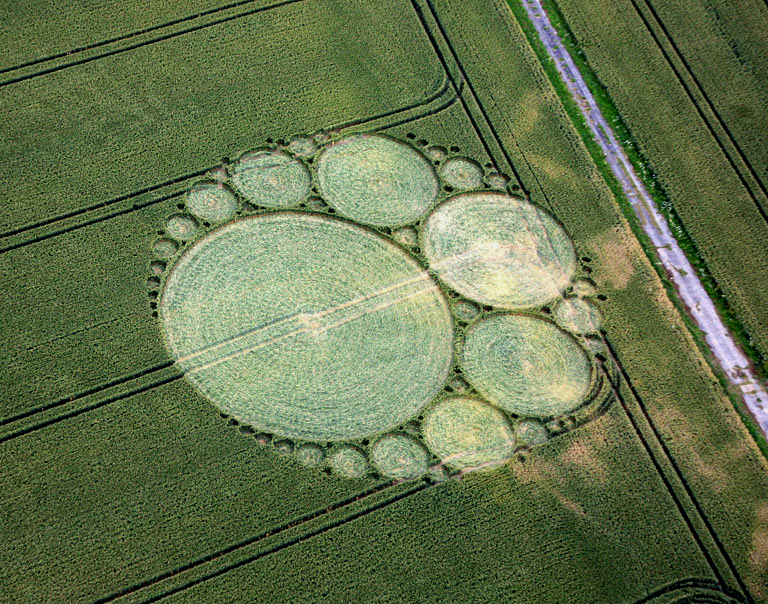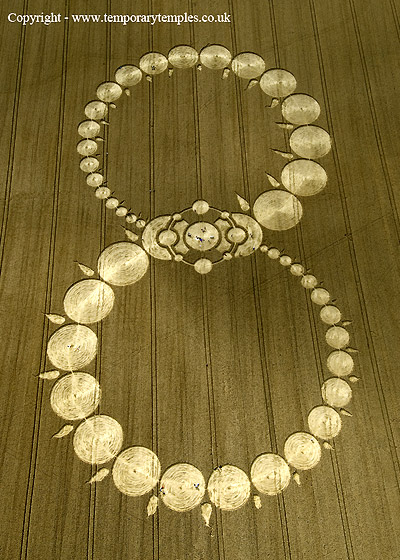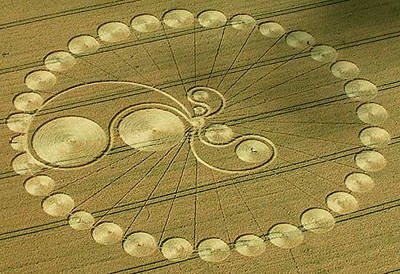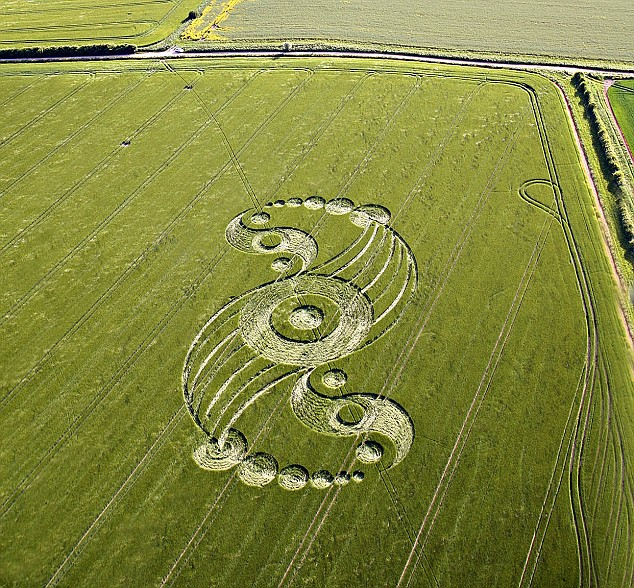# Crop Circles Art: Apollonius Circles, Taiji Diagram

By Xah Lee. Date:

Circles touching circles is a common plane geometry problem. They are not as easy to design than grid patterns or radiating patterns, because the problem of finding the right circle center and size takes some advanced plane geometry to known. See: [ Problem of Apollonius ] [ https://en.wikipedia.org/wiki/Problem_of_Apollonius ].

## Touching CirclesThis one is called [ Apollonian gasket ] [ https://en.wikipedia.org/wiki/Apollonian_gasket ]. image sourceIn this one, you have tangent circles around 2 large circles, with the tangent circles increasing in size. Once you decided that you want n circles to go around, the problem is how do you determine their center and radius. This would be a interesting exercise.

## Taiji Diagram DesignsIn this design, it is made of 3 taiji diagrams of different sizes and positions. The taiji diagram ☯ , aka Yin Yang symbol, is a simple circle composition.This design is another variation based on the taiji diagram design. Instead of using 2 circles, it uses 3. [see Geometry: Transformation of the Plane II]